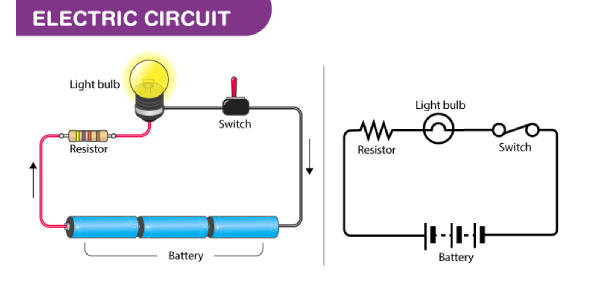# Electrical Circuit Test! Trivia Questions Quiz

15 Questions | Attempts: 444
ShareSettings.

• 1.
Which of the following describes a series circuit?
• A.

Current flows along each branch

• B.

Bulbs are all in a loop

• C.

If one bulb breaks the other one still works

• 2.
Which of the following describes a parallel circuit?
• A.

All bulbs light up together

• B.

If one bulb breaks the circuit is broken

• C.

Current flows along each branch

• 3.
What units does the electrical current have?
• A.

Amps

• B.

Volts

• C.

Power

• 4.
What is the symbol for a bulb or lamp?
• A.

A rectangle

• B.

A circle with an x

• C.

One big and one small line

• D.

A bulb shape with a coil inside

• 5.
What type of circuit do we find in our houses?
• A.

Parallel

• B.

Series

• 6.
When you move your brain uses electrical signals; these are called:
• A.

Nerve signals

• B.

Electrical signals

• C.

Impulses

• 7.
If your heart stops, doctors can try to start it again by using a
• A.

Electric shocker

• B.

Defibrillator

• C.

Pacemaker

• 8.
When your skin is wet, electricity can pass through you
• A.

More easily

• B.

Harder

• C.

About the same as dry skin

• 9.
"In parallel" means that a circuit has:
• A.

One path

• B.

Two paths

• C.

Multiple paths

• D.

All of the above

• 10.
What is the name of the law that keeps track of volts, ohms, and amperes?
• A.

Newton's Law Pascal's Law

• B.

Kirchoff's Law

• C.

Coulomb's Law

• D.

Ohm's Law

• 11.
When measuring ohms in a circuit, you are measuring:
• A.

Current

• B.

Voltage

• C.

Resistance

• D.

Power

• 12.
Variable resistors are called:
• A.

Rheostats

• B.

Trimmers

• C.

Potentiometers

• D.

All of the above

• 13.
How can you determine the value of a resistor?
• A.

Weigh the resistor

• B.

• C.

Check the voltage

• D.

None of the above

• 14.
The formula RT = R1 + R2 + R3 indicates:
• A.

An open circuit

• B.

A short circuit

• C.

A parallel circuit

• D.

A series circuit

• 15.
Direct current is current that flows
• A.

In two directions

• B.

In one direction only

• C.

Up and down

• D.

In pulses

## Related TopicsBack to top
×

Wait!
Here's an interesting quiz for you.